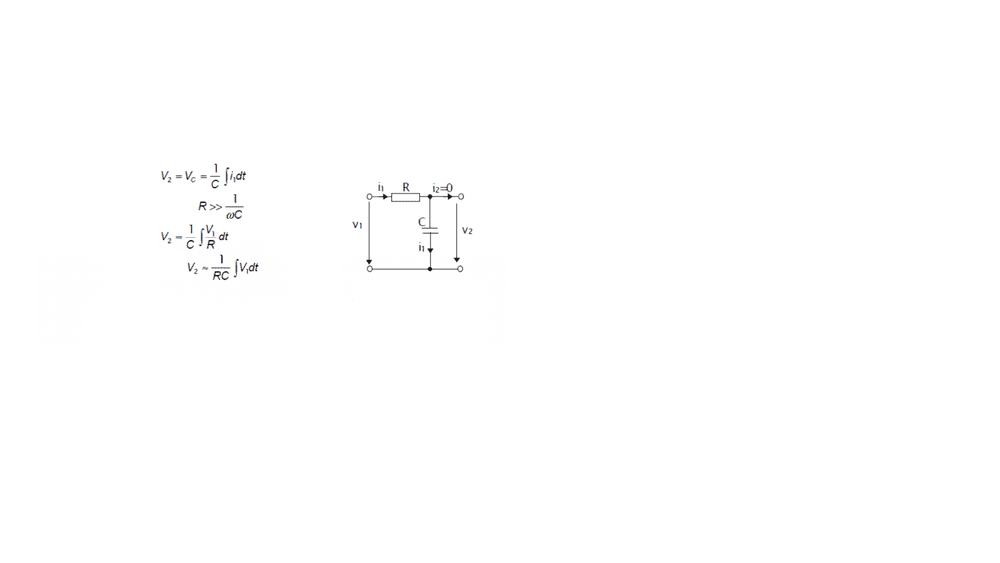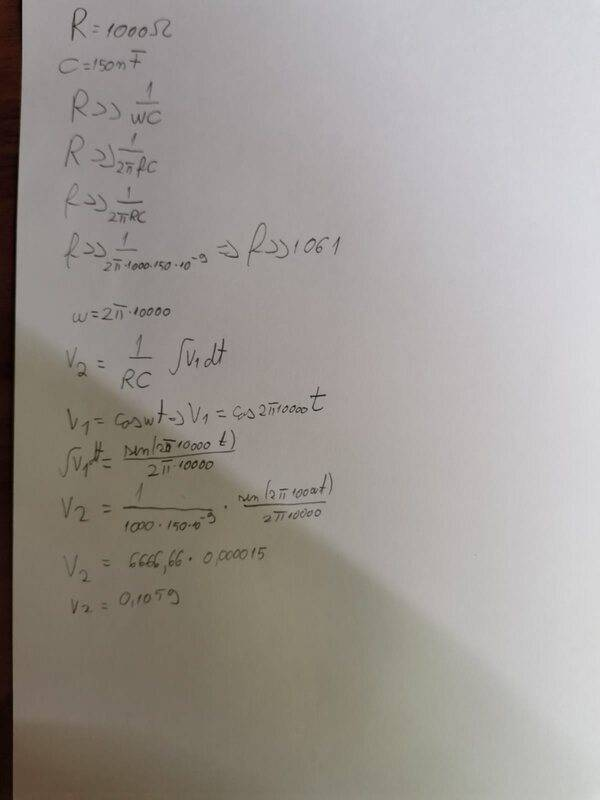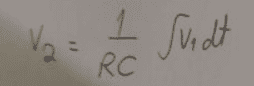# RC integrator circuit

• Engineering
hmzi123
Homework Statement:
RC integrator circuit
Relevant Equations:
RC integrator circuitsHello, i am student from Romania ( first year) and my physics teacher told us to solve this problem. We know that C=150 nanofarad, R= 1000 ohms, and the frequency we should take is more then 1061 ( 10kHz, to be more exactly). My problem is that i don't know what to take as V1 ( i took it coswt, where w=2*pi*10000), can you help me understand how to choose the V1, and what its formula is so that I can calculate and find V2.

Last edited:

Homework Helper
Hello hm,!

told us to solve this problem
Did you forget to mention the 'problem'? I don't see what is asked !

hmzi123
Hello hm,!

Did you forget to mention the 'problem'? I don't see what is asked !
The value of V2. Sorry!

Homework Helper
No need to apologize. Can you render the complete problem statement ? Asking for V2 when nothing is given for V1 is weird. The picture does not help much either. The only thing I can suppose is that you are asked to derive ##V_2\; {\bf \approx}\; \displaystyle {1\over RC}\int V_1 dt\quad ## i.e. show what is small enough to be ignored wrt what else.

hmzi123
No need to apologize. Can you render the complete problem statement ? Asking for V2 when nothing is given for V1 is weird. The picture does not help much either. The only thing I can suppose is that you are asked to derive ##V_2\; {\bf \approx}\; \displaystyle {1\over RC}\int V_1 dt\quad ## i.e. show what is small enough to be ignored wrt what else.
I took v1 as coswt where w is the 2*pi*frequency ( in this case the teacher said to pick the frequency 10000). Is this correct as a formula for v1 or not?

Homework Helper
It satisfies ##R >> {1\over \omega C}##, so yes.

 actually more ##R > {1\over \omega C}## but you can calculate that for yourself, can't you.

Can you find out why it says ##\ \approx\ ## and not ##\ =\ ## ?

Gold Member
Can you find out why it says ##\ \approx\ ## and not ##\ =\ ## ?
It should be ##\approx## there and the line above it (if I'm seeing that tiny font correct) because ##i \neq V_1/R##. They're omitting the small impedance from the capacitor, which is being dominated by a much larger ##R##.

Another question is why not solve this in frequency domain? Has that been covered in the class?

hmzi123After solving this is what i got. I know i should calculate v2 max value so where i wrote sin(2pi*10000t) i thought that the max value of any sin is 1 so that means the fraction will be 1/(2*pi*10000). Did i solve this any good?